# Surface area + Pythagorean theorem - math problems

#### Number of problems found: 167

• School modelThe beech school model of a regular quadrilateral pyramid has a base 20 cm long and 24 cm high. Calculate a) the surface of the pyramid in square decimeters, b) the mass of the pyramid in kilograms if the density of the beech is ρ = 0,8 g/cm ^ 3
• Right-angled triangle baseFind the volume and surface area of a triangular prism with a right-angled triangle base if the length of the prism base legs are 7.2 cm and 4.7 cm and the height of a prism is 24 cm.
• How toHow to find a total surface of a rectangular pyramid if each face is to be 8 dm high and the base is 10 dm by 6 dm.
• Frustrum - volume, areaCalculate the surface and volume of the truncated cone, the radius of the smaller figure is 4 cm, the height of the cone is 4 cm and the side of the truncated cone is 5 cm.
• Regular square prismThe volume of a regular square prism is 192 cm3. The size of its base edge and the body height is 1: 3. Calculate the surface of the prism.
• Axial sectionCalculate the volume and surface of a cone whose axial section is an equilateral triangle with side length a = 18cm.
• The tetrahedron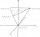Calculate the surface area and volume of a regular tetrahedron 4.9 cm high, the base edge has a length of 6 cm.
• Martians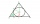A sphere-shaped spaceship with a diameter of 6 m landed in the meadow. In order not to attract attention, the Martians covered it with a roof in the shape of a regular cone. How high will this roof be so that the consumption of roofing is minimal?
• Base diagonalIn a regular 4-sided pyramid, the side edge forms an angle of 55° with the base's diagonal. The length of the side edge is eight meters. Calculate the surface area and volume of the pyramid.
• Side edgesThe regular 4-sided pyramid has a body height of 2 dm, and the opposite side edges form an angle of 70°. Calculate the surface area and volume of the pyramid.
• Surface and volume - cubeFind the surface and volume of a cube whose wall diagonal is 5 cm long.
• The rotating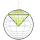The rotating cone has a height of 0.9 m and the diameter of the base is 7.2 dm. Calculate the surface of the cone. (Hint: use Pythagorean theorem for a side of cone)
• The volumeThe volume of the cone is 94.2dm³, the radius of the base is 6 dm Calculate the surface of the cone.
• Truncated pyramidFind the volume and surface area of a regular quadrilateral truncated pyramid if base lengths a1 = 17 cm, a2 = 5 cm, height v = 8 cm.
• A spherical segment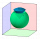A spherical section whose axial section has an angle of j = 120° in the center of the sphere is part of a sphere with a radius r = 10 cm. Calculate the cut surface.
• Pentagonal pyramidThe height of a regular pentagonal pyramid is as long as the edge of the base, 20 cm. Calculate the volume and surface area of the pyramid.
• Truncated cone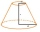Find the volume and surface area of the truncated cone if r1 = 12 cm, r2 = 5 cm and side s = 10 cm.
• Regular 4-sided pyramidFind the area (surface area) of a regular 4-sided pyramid if its height is 20 m and the wall height is 23 m.
• The cone - S,VCalculate the volume and surface area of the cone if its radius r = 6 cm and side s = 10 cm.
• Průměr kuželeVypočtěte povrch a objem rotačního kužele jehož průměr je 60mm a délka strany 3.4 cm.

Do you have an exciting math question or word problem that you can't solve? Ask a question or post a math problem, and we can try to solve it.

We will send a solution to your e-mail address. Solved examples are also published here. Please enter the e-mail correctly and check whether you don't have a full mailbox.

Please do not submit problems from current active competitions such as Mathematical Olympiad, correspondence seminars etc...

Pythagorean theorem is the base for the right triangle calculator. Examples for the calculation of the surface area of ​​the solid object . Pythagorean theorem - math problems.